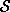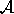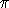## CGAL::snap_rounding_2

### Definition

Snap Rounding (SR, for short) is a well known method for converting arbitrary-precision arrangements of segments into a fixed-precision representation [GGHT97, GM98, Hob99]. In the study of robust geometric computing, it can be classified as a finite precision approximation technique. Iterated Snap Rounding (ISR, for short) is a modification of SR in which each vertex is at least half-the-width-of-a-pixel away from any non-incident edge [HP02]. This package supports both methods. Algorithmic details and experimental results are given in [HP02].

Given a finite collectionof segments in the plane, the arrangement ofdenoted() is the subdivision of the plane into vertices, edges, and faces induced by. A vertex of the arrangement is either a segment endpoint or the intersection of two segments. Given an arrangement of segments whose vertices are represented with arbitrary-precision coordinates, SR proceeds as follows. We tile the plane with a grid of unit squares, pixels, each centered at a point with integer coordinates. A pixel is hot if it contains a vertex of the arrangement. Each vertex of the arrangement is replaced by the center of the hot pixel containing it and each edge e is replaced by the polygonal chain through the centers of the hot pixels met by e, in the same order as they are met by e.

In a snap-rounded arrangement, the distance between a vertex and a non-incident edge can be extremely small compared with the width of a pixel in the grid used for rounding. ISR is a modification of SR which makes a vertex and a non-incident edge well separated (the distance between each is at least half-the-width-of-a-pixel). However, the guaranteed quality of the approximation in ISR degrades. For more details on ISR see [HP02].

The traits used here must support (arbitrary-precision) rational number type as this is a basic requirement of SR.

#include <CGAL/Snap_rounding_2.h>

template < class Traits, class InputIterator, class OutputContainer >
void
 snap_rounding_2 ( InputIterator begin, InputIterator end, OutputContainer& output_container, typename Traits::FT pixel_size, bool do_isr = true, bool int_output = true, unsigned int number_of_kd_trees = 1)

The first two parameters denote the first and after-the-last iterators of the input segments. The third parameter is a reference to a container of the output polylines. Since a polyline is composed of a sequence of points, a polyline is a container itself. The fifth parameter determines whether to apply ISR or SR.

The forth parameter denotes the pixel size w. The plane will be tiled with square pixels of width w such that the origin is the center of a pixel. The sixth parameter denotes the output representation. If the value of the sixth parameter is true then the centers of pixels constitute the integer grid, and hence the vertices of the output polylines will be integers. For example, the coordinates of the center of the pixel to the right of the pixel containing the origin will be (1,0) regardless of the pixel width. If the value of the sixth parameter is false, then the centers of hot pixels (and hence the vertices of the output polylines) will bear their original coordinates, which may not necessarily be integers. In the latter case, the coordinates of the center of the pixel to the right of the pixel containing the origin, for example, will be (w,0).

The seventh (and last) parameter is briefly described next; for a detailed description see [HP02].advancedA basic query used in the algorithm is to report the hot pixels of size w that a certain segment s intersects. An alternative way to do the same is to query the hot pixels' centers contained in a Minkowski sum of s with a pixel of width w centered at the origin; we denote this Minkowski sum by M(s). Since efficiently implementing this kind of query is difficult, we use an orthogonal range-search structure instead. We query with the bounding box B(M(s)) of M(s) in a two-dimensional kd-tree which stores the centers of hot pixels. Since B(M(s)) in general is larger than M(s), we still need to filter out the hot pixels which do not intersect s.

While this approach is easy to implement with CGAL, it may incur considerable overhead since the area of B(M(s)) may be much larger than the area of M(s), possibly resulting in many redundant hot pixels to filter out. Our heuristic solution, which we describe next, is to use a cluster of kd-trees rather than just one. The cluster includes several kd-trees, each has the plane, and hence the centers of hot pixels, rotated by a different angle in the first quadrant of the plane; for our purpose, a rotation by angles outside this quadrant is symmetric to a rotation by an angle in the first quadrant.

Given a parameter c, the angles of rotation are (i - 1) ()/(2c), i=1,...,c, and we construct a kd-tree corresponding to each of these angles. Then for a query segment s, we choose the kd-tree for which the area of B(M(s)) is the smallest, in order to (potentially) get less hot pixels to filter out. Since constructing many kd-trees may be costly, our algorithm avoids building a kd-tree which it expects to be queried a relatively small number of times (we estimate this number in advance). How many kd-trees should be used? It is difficult to provide a simple answer for that. There are inputs for which the time to build more than one kd-tree is far greater than the time saved by having to filter out less hot pixels (sparse arrangements demonstrate this behavior), and there are inputs which benefit from using several kd-trees. Thus, the user can control the number of kd-trees with the parameter number_of_kd_trees. Typically, but not always, one kd-tree (the default) is sufficient.advancedPrecondition

pixel_size must have a positive value and number_of_kd_trees must be a positive integer.